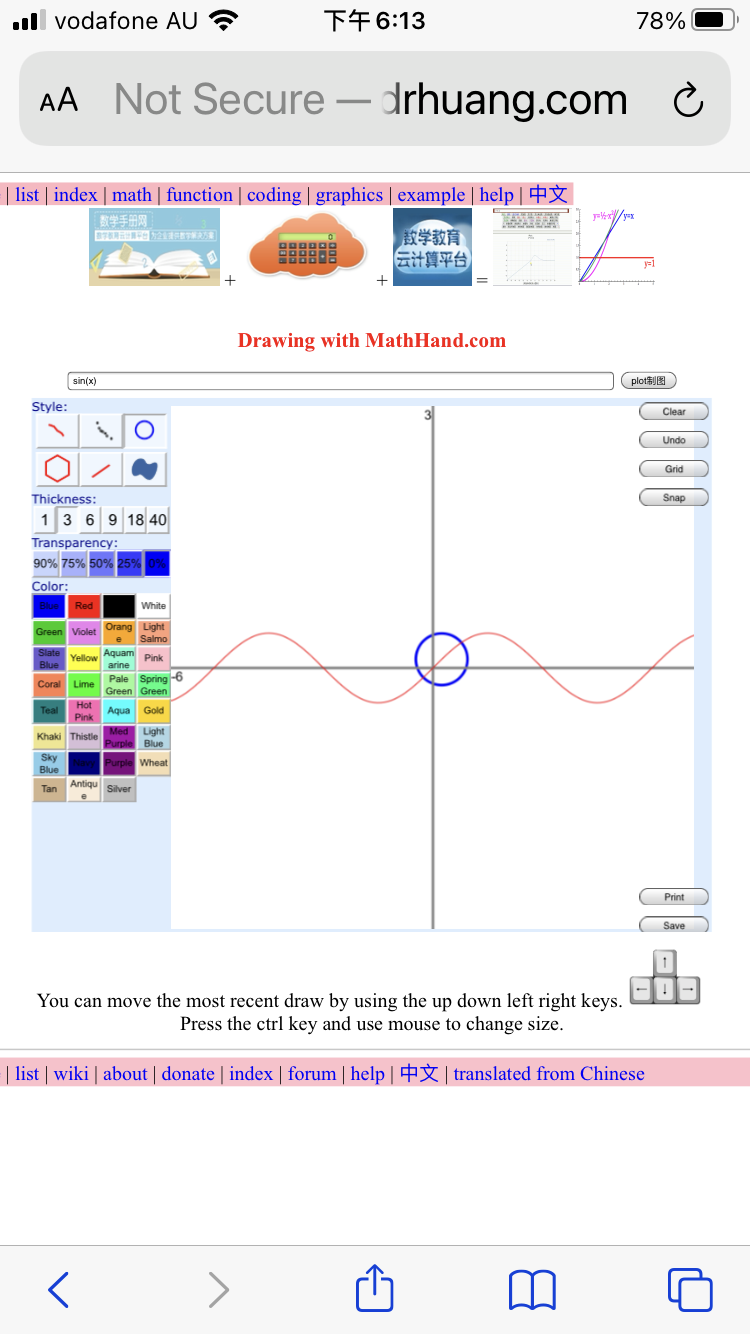﻿ ﻿ Fractional Calculus Computer Algebra System, Electrochemistry Software, chat GPT+++=# Example of Graphics 制图

## Content

1. Classification by plotting function 按制图函数分类
1. plot(x) 制图
2. polarplot(x) 极坐标图
3. complexplot(z) 复变函数图
4. complex2D(x) 2 Curve 复函数2D
5. plot2D(x) 制图2D
6. Webpage editor
7. surface3D(x) 表面图3D
8. function3D(x) 函数图3D
9. plot3D(x) 立体图3D
10. section3D(x) 截面图3D
11. spin3D(x) 旋转图3D
12. contour3D(x) 线框图3D
13. complex3D(x) 复变函数图3D
14. parametric3D(t,t,t) 参数立体图3D
15. wireframe3D(x) 立体线框图3D
16. implicit3D(x-y-z) 隐函数图3D
17. math3D 数学图3D
18. data3D 数据3D图
2. Classification by Dimension 按维数分类
1. plane curve 2D 平面图
1. important plane curve
2. Interactive plot with plot(x) 互动制图
3. parametric plot wth parametricplot(t,t)
4. polar plot with polarplot(x)
5. solve equation graphically with plot( )
6. dynamic plot with tangentplot(x) 制图
7. complex plot with complexplot(z)
8. Geometry 几何 with circle(1)
9. plane graph 平面图 with plot2D(x)
10. function plot with funplot(x)
11. differentiate graphically with diff2D(x)
12. integrate graphically with integrate2D(x)
13. solve ODE graphically with odeplot(y'=x)
14. animation with timer(1)
2. surface 2D
3. space curve 3D
4. surface 3D
5. surface 4D equation
3. Classification by appliaction 按应用分类
4. Classification by objects 按物体分类
5. Classification by function 按函数分类
6. Classification by equation 按方程分类
7. Classification by domain 按领域分类
8. Classification by libary 按文库分类
9. Classification by platform 按平台分类

## Complex

1. complex - complex function - complex math
2. math handbook chapter 10 complex function
3. complex animate(z) for phase animation, the independent variable must be z.
4. complex plot(z) for phase and/or modulus, the independent variable must be z.
5. complex2D(x) for complex 2 curves of real and imag parts, the independent variable must be x.
6. complex3D(x) for 3 dimensional graph, the independent variable must be x.
7. color WebXR surface of complex function on complex plane
8. Riemann surface - Complex Branches - complex coloring

## Credit

Paul Masson, Xiao Gang, Theo Armour, Christopher Chudzicki,